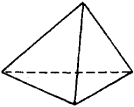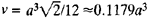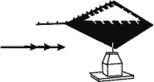# tetrahedron

(redirected from 3-demihypercube)
Also found in: Dictionary, Thesaurus.

## tetrahedron:

see polyhedronpolyhedron
, closed solid bounded by plane faces; each face of a polyhedron is a polygon. A cube is a polyhedron bounded by six polygons (in this case squares) meeting at right angles.
.

## Tetrahedron

A polygon with four plane surfaces.
The following article is from The Great Soviet Encyclopedia (1979). It might be outdated or ideologically biased.

## Tetrahedron

(or triangular pyramid), a polyhedron with four triangular faces, six edges, and four vertices. At each vertex three edges intersect. The regular tetrahedron (Figure 1) is oneFigure 1

of the five regular polyhedrons. If a is the length of an edge of a regular tetrahedron, the volume of the tetrahedron is.

## tetrahedron

[‚te·trə′hē·drən]
(crystallography)
An isometric crystal form in cubic crystals, in the shape of a four-faced polyhedron, each face of which is a triangle.
(mathematics)
A four-sided polyhedron.
McGraw-Hill Dictionary of Scientific & Technical Terms, 6E, Copyright © 2003 by The McGraw-Hill Companies, Inc.

## tetrahedronA device to indicate wind direction, and, in turn, landing direction. It is tetrahedronshaped—four triangular sides. This device is generally located at uncontrolled airports. The small end of a tetrahedron points in the direction of landing. At controlled airports, the tetrahedron should be disregarded because tower instructions supercede the indicator. On approach charts, a tetrahedron is shown as.